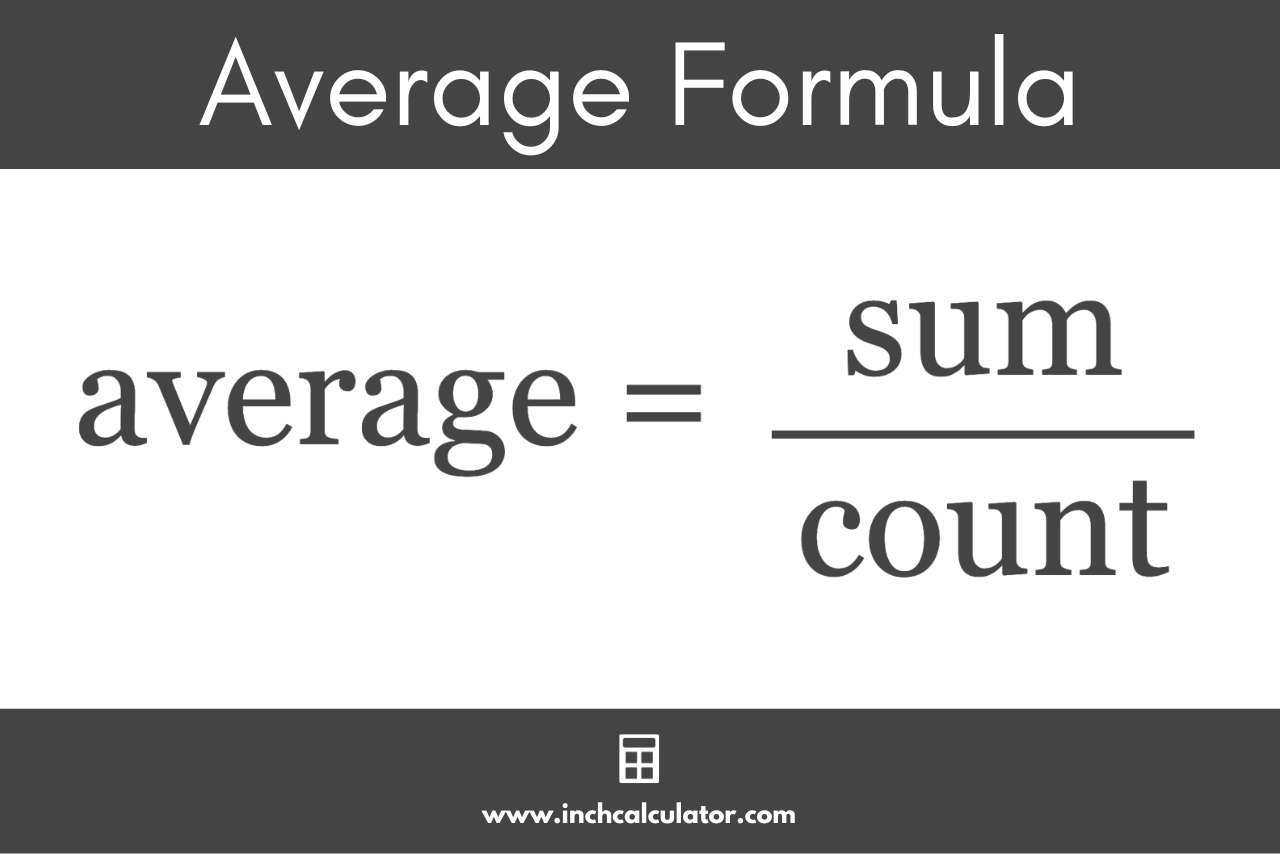# Average Calculator

Find the average value by entering the numbers below.

Separate numbers using a comma (,)

## Average:

### Steps to Solve

count = ?

sum = ?

#### Step Three: Use the Average Formula

average = sum / count

Learn how we calculated this below

## How to Find the Average

The average, or mean, is a measure of the central tendency of a set of numbers. It is a single number used to represent the center, or typical value, for a larger set of numbers.

The average is one of the fundamental measurements for a data set in statistics and is used as the basis for several other measurements.

There are several types of averages that include arithmetic averages, geometric averages, weighted averages, and even harmonic averages. The calculator above solves the arithmetic average, also called the arithmetic mean.

You can find the mean using a formula.

### Average Formula

The average formula to find the mean for a set of numbers is:

average = sum / count

The average of a set of numbers is equal to the sum of the numbers divided by the count of the numbers.### Find the Average in Three Easy Steps

So, you can find the average using the formula above in three steps.

#### Step One: Find the Count

The first step to find the average is to count the number of values in the data.

#### Step Two: Find the Sum

The second step is to add all of the numbers together to calculate the total sum of the numbers.

#### Step Three: Find the Average

The final step is to use the average formula above by dividing the sum of the numbers by the count. The resulting value is the average of the data.

While the average, or mean, is commonly used, it is just one measure of the center for a data set. Other commonly used measures of center are the median and mode.

You can also use our mean, median, mode calculator to find other measures of center for your data.

These other measurements are typically used since the average measurement can be easily skewed by outliers (extreme values) in the data.

But, the median and mode of your grades is an A since they are less skewed by one grade in the whole set, which is an extreme outlier.

### What's an average in math?

The average is the measure of the central tendency, or the middle, of a set of numbers.

### Does average always mean the mean?

Yes, the average is the same as the arithmetic mean.

### Which is better, average or median?

Neither the average or median is better than the other. The average works well with data that does not contain many outliers, but the median works well with data that contains outliers.

### What does it mean if average is higher than median?

If the average is higher than the median, that means that your data is skewed higher by one or more outliers in the data.